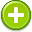#likelihood 中文解釋 wordnet sense Collocation Usage
Noun
/ˈlīklēˌho͝od/,Font size:The state or fact of something's being likely; probability,
1. The state or fact of something's being likely; probability
• - young people who can see no likelihood of finding employment
• - situations where there is a likelihood of violence

1. the probability of a specified outcome
2. In statistics, the likelihood function (often simply the likelihood) is a function of the parameters of a statistical model that plays a key role in statistical inference. ...
3. Given a hypothesis, the probability of observing certain data.
4. The possibility that a given event will occur.
5. The conditional probability of observable evidence given a hypothesized state and set of parameters. This is often written where represents the observable evidence, and is a parameter or unknown variable. ...
6. A qualitative description of a probability or frequency.
7. is the probability density of observations calculated from parameters and not observations.
8. promise, appearance.